# Review — Seq2Seq: Sequence to Sequence Learning with Neural Networks

## Using LSTM for Encoder and Decoder for Machine Translation

In this story, Sequence to Sequence Learning with Neural Networks, by Google, is reviewed. In this paper:

• A multilayered Long Short-Term Memory (LSTM) to map the input sequence to a vector of a fixed dimensionality, and then another deep LSTM to decode the target sequence from the vector.
• This general end-to-end approach to sequence learning, improves the statistical machine translation (SMT) e.g.: English to French translation task.

This is a paper in 2014 NeurIPS with over 16000 citations. (Sik-Ho Tsang @ Medium) Though many people have already known this paper, this is my first Medium story about NLP.

# Outline

1. The Use of LSTM
2. Sequence to Sequence (Seq2Seq) Model
3. Experimental Results

# 1. The Use of LSTM

• The Recurrent Neural Network (RNN) is a natural generalization of feedforward neural networks to sequences. Given a sequence of inputs (x1, ..., xT), a standard RNN computes a sequence of outputs (y1, …, yT) by iterating the following equation:
• However, it is difficult to train the RNNs due to the resulting long term dependencies. Gradient vanishing problem occurs in vanilla RNN when the sentence is too long.

LSTM is used to tackle the gradient vanishing problem.

# 2. Sequence to Sequence (Seq2Seq) Model

## 2.1. Framework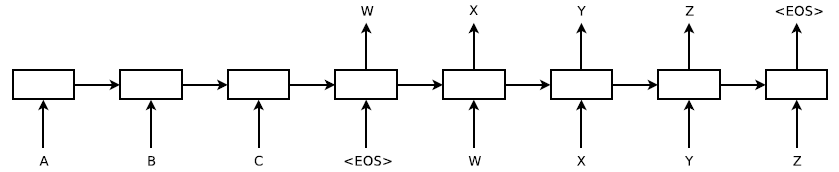Sequence to Sequence (Seq2Seq) Model. The Seq2Seq model reads an input sentence “ABC” and produces “WXYZ” as the output sentence.
• A straightforward application of the Long Short-Term Memory (LSTM) architecture can solve general sequence to sequence problems.
• The idea is to use one LSTM to read the input sequence, one timestep at a time, to obtain large fixed-dimensional vector representation;
• and then to use another LSTM to extract the output sequence from that vector . The second LSTM is essentially a recurrent neural network language model except that it is conditioned on the input sequence.
• Thus, the goal of the LSTM is to estimate the conditional probability p(y1, ..., yT′ | x1, ..., xT).

The LSTM computes this conditional probability by first obtaining the fixed-dimensional representation v of the input sequence (x1, …, xT) given by the last hidden state of the LSTM, and then computing the probability of y1, …, yT′ with a standard LSTM-LM (Language Model) formulation whose initial hidden state is set to the representation v of x1, …, xT:

In addition, the LSTM reads the input sentence in reverse, because doing so introduces many short term dependencies in the data that make the optimization problem much easier.

## 2.2. Training

• The core of the experiments involved training a large deep LSTM on many sentence pairs. Seq2Seq is trained by maximizing the log probability of a correct translation T given the source sentence S, so the training objective is:
• where S is the training set.
• Deep LSTMs with 4 layers are trained, with 1000 cells at each layer and 1000 dimensional word embeddings, with an input vocabulary of 160,000 and an output vocabulary of 80,000.
• It is found that deep LSTMs to significantly outperform shallow LSTMs, where each additional layer reduced perplexity by nearly 10%, possibly due to their much larger hidden state.
• A naive softmax is used over 80,000 words at each output.
• The resulting LSTM has 380M parameters of which 64M are pure recurrent connections (32M for the “encoder” LSTM and 32M for the “decoder” LSTM).
• Batches of 128 sequences are used.
• A hard constraint is applied on the norm of the gradient [10, 25] to prevent from gradient explosion.

## 2.3. Testing

• Once training is complete, translations are produced by finding the most likely translation according to the LSTM:
• The most likely translation is searched using a simple left-to-right beam search decoder which maintains a small number B of partial hypotheses.
• At each timestep we extend each partial hypothesis in the beam with every possible word in the vocabulary. This greatly increases the number of the hypotheses so all are discarded but only the B most likely hypotheses are remained according to the model’s log probability.
• As soon as the “<EOS>” symbol is appended to a hypothesis, it is removed from the beam and is added to the set of complete hypotheses.
• Interestingly, Seq2Seq performs well even with a beam size of 1, and a beam of size 2 provides most of the benefits of beam.

# 3. Experimental Results

## 3.1. WMT’14 English to French test set (ntst14)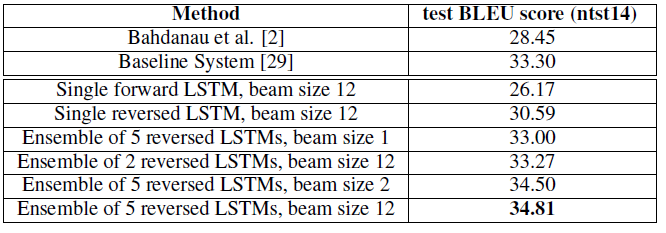The performance of the LSTM on WMT’14 English to French test set (ntst14)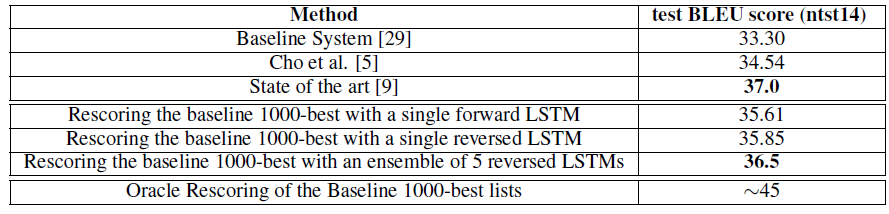Methods that use neural networks together with an SMT system on the WMT’14 English to French test set (ntst14)
• (For more details about BLEU: Please feel free to watch: C5W3L06 Bleu Score (Optional))
• The best results are obtained with an ensemble of LSTMs that differ in their random initializations and in the random order of minibatches.

It is the first time that a pure neural translation system outperforms a phrase-based SMT baseline on a large MT task by a sizeable margin, despite its inability to handle out-of-vocabulary words.

• The LSTM is within 0.5 BLEU points of the previous state of the art by rescoring the 1000-best list of the baseline system.

## 3.2. Long Sentences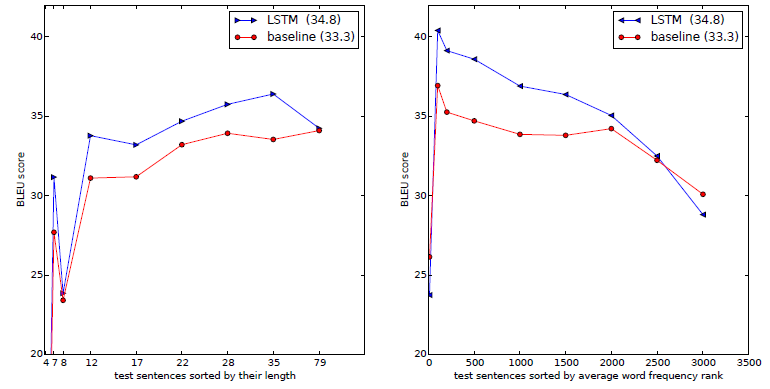Left: Performance of our system as a function of sentence length, Right: the LSTM’s performance on sentences with progressively more rare words
• Left: There is no degradation on sentences with less than 35 words, there is only a minor degradation on the longest sentences.
• Right: Better BLEU for rarely appeared words.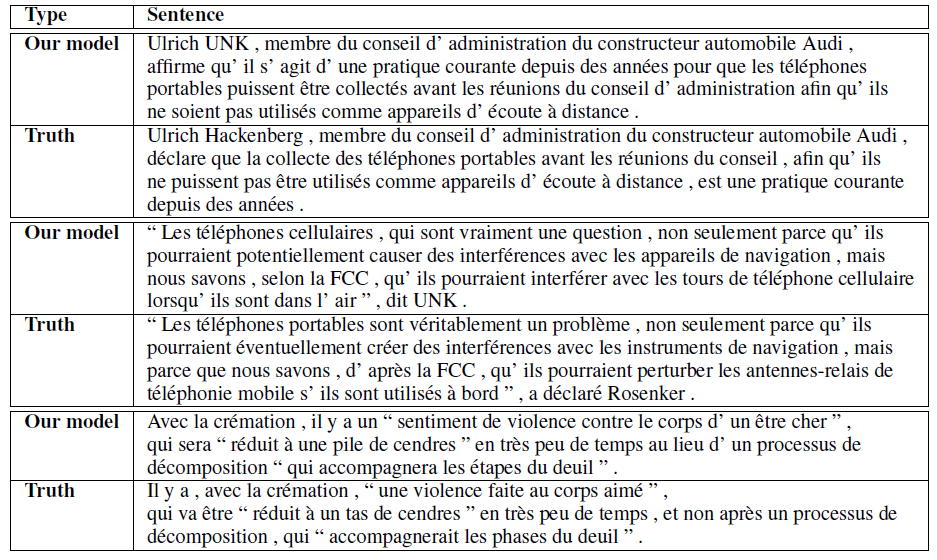A few examples of long translations produced by the LSTM alongside the ground truth translations
• The reader can verify that the translations are sensible using Google translate.

## 3.3. Model Analysis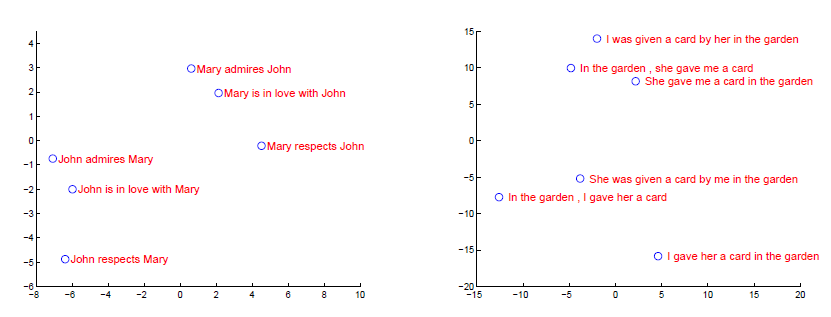The figure shows a 2-dimensional PCA projection of the LSTM hidden states that are obtained after processing the phrases in the figures
• The above figure clearly shows that the representations are sensitive to the order of words, while being fairly insensitive to the replacement of an active voice with a passive voice.

This is one of the beginner papers for Natural Language Processing (NLP). NLP techniques are useful for the data that related to time or data with sequential order.

## Reference

[2014 NeurIPS] [Seq2Seq]
Sequence to Sequence Learning with Neural Networks

2014 [Seq2Seq]

--

--